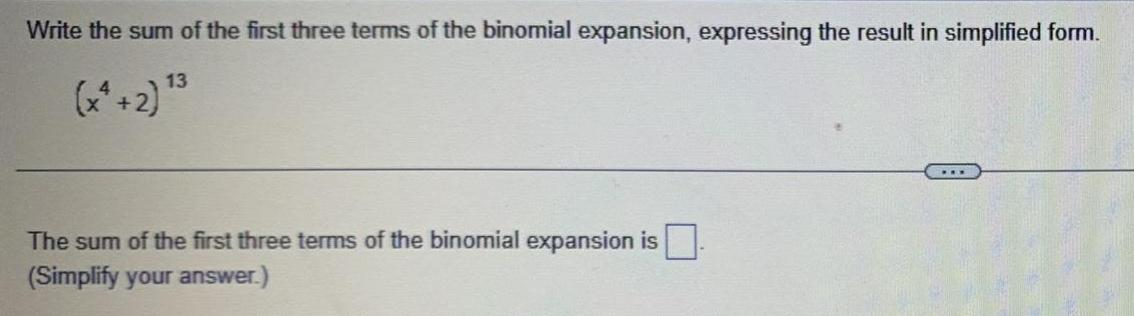Question:

# Write the sum of the first three terms of the binomial

Last updated: 11/17/2023Write the sum of the first three terms of the binomial expansion expressing the result in simplified form 13 x 2 The sum of the first three terms of the binomial expansion is Simplify your answer